## Monday, March 31, 2014 ...//### BICEP is good news for string gas cosmology

Guest post by Robert Brandenberger of McGill UniversityThe indirect discovery of primordial gravitational waves on cosmological scales via B-mode polarization of the cosmic microwave background is being interpreted by most people inside and outside of the cosmology community in the context of the inflationary universe scenario. The purpose of this guest blog is to remind the readers that gravitational waves on cosmological scales is a prediction which is not unique to inflationary cosmology. In particular, "String Gas Cosmology", a scenario initially proposed in 1989 for the early universe and which is based on fundamental principles of superstring theory predicts a spectrum of gravitational waves with significant amplitude. In this blog I will briefly explain the scenario and its predictions. Specifically, String Gas Cosmology predicts a slightly blue spectrum of gravitational waves, a prediction which allows us to differentiate the scenario from inflationary cosmology which generically predicts a tilt of the spectrum which is slightly red.

BICEP results

The BICEP-2 results announced a week ago are wonderful news for early universe cosmologists. They provide an indirect discovery of gravitational waves with wavelengths close to the radius of the currently visible universe. These waves are almost without doubt produced in the very early universe, and thus provide us with a tool to explore the physics of the very early universe.

As Liam McAllister has emphasized in his guest blog, what has been discovered by the BICEP collaboration is B-mode polarization in the cosmic microwave background (CMB). At this stage it is important to remain a bit cautious. It is very difficult to experimentally make sure that no signal of the much larger amplitude E-mode polarization mode is misidentified as a contribution to the B-mode. Hence, it is crucial for other experiments to confirm the BICEP results. However, let us assume now that the results will stand up against further scrutiny.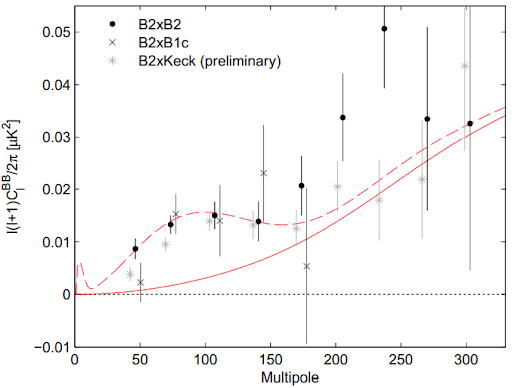Figure 1: The BB auto and cross correlation functions as seen by the BICEP collaboration (courtesy of BICEP). The vertical axis gives the amplitude of the correlation, the horizontal axis represents angular scale (large angles on the left, small angles on the right).
The BICEP-2 paper reports the angular power spectrum of B-mode polarization on intermediate angular scales, in particular on scales where the contribution to the spectrum from gravitational lensing of the E-mode polarization (measured recently by the South Pole Telescope and the Polarbear collaborations) falls off and is predicted to be smaller than the primordial B-mode signal produced in the early universe. The amplitude of the gravitational waves is higher than many theorists expected based on recent results of CMB temperature measurements by the Planck satellite. In addition, the spectral shape appears to be slightly shifted to the blue compared to a scale-invariant one, which means that there is more power on shorter wavelengths than longer ones (see Figure 1). Whereas the statistical significance of the detection of a large amplitude of gravitational waves is high, the significance of the results on the tilt is still marginal.

Gravitational Waves from Early Universe Cosmology

Most cosmologists are interpreting the results in the framework of the inflationary universe scenario, our current paradigm for understanding the early universe. As Liam reviewed in his guest blog, the inflationary scenario of the early universe can solve a number of important puzzles which Standard Big Bang cosmology faced, in particular the "horizon problem", the mystery surrounding the origin of the overall isotropy of the observed. Inflation solves this problem by postulating a period of nearly exponential expansion of space which inflates the initial causal patch to distances much larger than those probed in cosmological observations. Inflationary cosmology also provided the first mechanism based on causal physics which leads small amplitude inhomogeneities in the distribution of galaxies. More specifically, the spectrum of these inhomogeneities is predicted to be roughly scale-invariant. As first studied in the case of gravitational waves by Starobinsky and in the case of matter fluctuations by Mukhanov and Chibisov, these perturbations are generated from quantum vacuum fluctuations in an early phase during which the size of the universe is postulated to increase almost exponentially, and the wavelength of the fluctuations is stretched to be much larger than the Hubble radius (see below).

The conditions which are required for a successful theory which explains the origin of the structure we observe in galaxy surveys and cosmic microwave anisotropy maps were discussed by Sunyaev and Zeldovich and by Peebles and Yu a decade before the development of inflationary cosmology. Before discussing these conditions, I must remind the reader of the existence of two very different length scales in cosmology, firstly the horizon and secondly the Hubble radius. They happen to be identical in Standard Big Bang cosmology, which is what gives rise to the "horizon problem".

The horizon is the forward light cone from a fixed position on the initial time surface. It gives us the distance which light can travel from the initial time, the "Big Bang". The Hubble radius, in contrast, is a local concept: it is simply the inverse Hubble expansion rate. Whereas the horizon is the important scale when considerations of causality are discussed, it is the Hubble radius which determines how fluctuations in both matter and metric evolve. On length scales smaller than the Hubble radius the fluctuations oscillate almost like in flat space-time. On the other hand, on scales larger than the Hubble radius the wave oscillations freeze out and the fluctuations evolve as standing waves whose amplitude increases.

The Hubble radius is the limiting scale on which fluctuations can be generated by local causal physics. Hence, the three conditions to obtain a viable theory of cosmological structure formation is that
1. the horizon is much larger than the Hubble radius (as is required to solve the horizon problem),

2. scales which are observed today originate on sub-Hubble scales in the very early universe (in order than a causal generation mechanism is possible), and

3. that a mechanism producing roughly scale-invariant primordial fluctuations in the early universe exists. If these conditions are satisfied, then the fluctuations will lead to the phenomena which have now been confirmed observationally, namely acoustic oscillations in the angular power spectrum of CMB anisotropies, and baryon acoustic oscillations in the matter power spectrum.
The inflationary paradigm is the first model based on causal physics in which the above three conditions are realized, but it is not the only one. "String Gas Cosmology" is another model.

String gas cosmology

String Gas Cosmology (SGC) was initially proposed in 1989. It is a scenario of the evolution of the very early universe based on basic principles of superstring theory. Specifically, SGC is a scenario which makes use of the new degrees of freedom and new symmetries which distinguish particle-based theories from string theory. The space of states of string theory is much richer than that of elementary point particle theories. For examples, let us consider space to be toroidal. In this case, then in addition to the degrees of freedom (the "momentum modes") which describe the center of mass motion which are common to strings and point particles, strings have a tower of oscillatory modes whose number increases exponentially with energy. In addition, there are "winding modes" which can be viewed as characterizing the number of times which a string can wind a compact spatial section. For more general spatial geometries, there are a multitude of string and brane configurations, and the same role which winding strings play on a torus can be played by various wrapping branes.

The existence of the many new string and brane degrees of freedom which set string theory part from point particle theories will lead to a very different thermodynamic behavior of the very early universe. For simplicity, let us again take space to be toroidal. In this case, it is already the existence of oscillatory and winding modes which sets string theory apart from point particle theories in a crucial way. If we imagine a gas of closed strings in thermal equilibrium and start this box off with a large radius $$R$$$, then most of the energy will be in the momentum modes. As the box slowly shrinks in size, the energy of the momentum modes increases (since it scales as $$1/R$$$) and hence the temperature increase. However, as the temperature approaches a critical value called the "Hagedorn temperature", then the increasing energy density will lead, rather than to a further increase in the energy of the momentum modes (and hence to an increase in temperature), to the production of oscillatory modes. Hence, the temperature of a gas of closed strings remains bounded. In fact, if the size of space decreases below the string scale, then the winding modes become light, the energy of the system will flow into those modes, and the temperature will decrease. There is in fact an important stringy symmetry: physics at large values of $$R$$$is equivalent to physics at small $$R$$$. This is one aspect of the T-duality symmetry of string theory. Note that these results all can be derived from the thermodynamics of closed heterotic strings.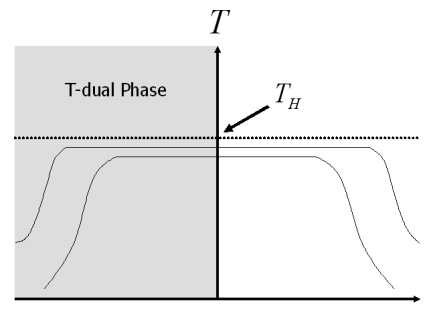Figure 2: Sketch showing how the temperature $$T$$$of a gas of strings (vertical axis) changes as a function of the size of space. The horizontal axis is logarithmic, with the center of the axis representing the string scale. Physics at small distances is equivalent to physics at large distances. The scaling of the temperature as a function of size is sketched in Figure 2. Note the broad plateau of values of $$R$$$ with almost constant temperature. The width of the plateau depends on the total entropy of space. Based on Figure 2 it is natural to expect that a state located in the plateau of Figure 2 will be quasi-static and long lived. Note that whereas this conclusion is very natural from the point of view of superstring theory, it is not from the point of view of a low energy effective field theory described by Einstein or dilaton gravity. But neither Einstein gravity nor dilaton gravity include the stringy symmetries which will determine the dynamics of the very early universe, and hence they are clearly not applicable. Note that the existence of a quasi-static equilibrium near the Hagedorn temperature is expected to be true generally by duality symmetries of strings, in a similar way to the "attractor mechanism" for black holes in string theory.

String Gas Cosmology thus leads to the following cosmologial background (once again, for simplicity, the dynamics is described in the case of a toroidal geometry): the universe lives for a long time in a quasi-static state at a temperature close to the Hagedorn temperature. The net winding charge of the string gas vanishes by symmetry. However, there are lots of strings which wind each of the nine spatial dimensions which exist. Eventually, due to the interactions of strings with opposite winding, three spatial dimensions can dynamically decompactify while the others care confined to the string scale forever. At this point our three spatial dimensions start to expand. The string unwindings provide a smooth transition from the early "Hagedorn phase" of string theory to the usual radiation phase of Standard Big Bang cosmology. The time evolution of the cosmological scale factor $$a(t)$$$(which gives the radius of spatial sections) is sketched in Figure 3.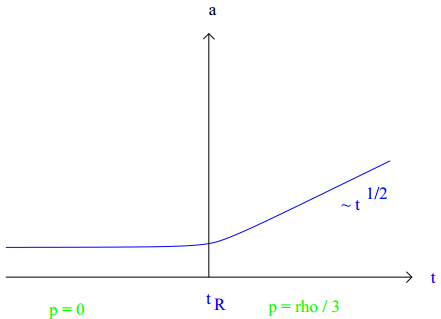Figure 3: Time evolution of the size of space in String Gas Cosmology. The vertical axis is proportional to the radius of space, the horizontal axis represents time. The time $$t_R$$$ is the end of the Hagedorn period.
String Gas Cosmology quite trivially satisfies the first two criteria for a successful early universe cosmology. This is illustrated in Figure 4 which shows the evolution of various physical wavelengths as a function of time (the vertical axis, with time $$t_R$$$denoting the end of the Hagedorn phase). The horizontal axis shows physical distance. During the Hagedorn phase, the Hubble radius is infinite because $$a(t)$$$ is constant. Once space starts to expand, the Hubble radius rather suddenly drops to a microsopic value at the beginning of the phase of Standard cosmology and from then on increases linearly in time as it does in Standard cosmology. The two thin lines which are vertical in the Hagedorn phase denote the physical wavelength of different fluctuation modes (the reader should visualize fluctuations in space as waves on the surface of a pond, the surface of the pond representing space). Once space begins to expand, the wavelength starts to increase in parallel. The thick curve denotes the Hubble radius. We thus see that
1. the horizon is much larger than the Hubble radius, demonstrating that String Gas Cosmology solves the Horizon Problem of Standard Big Bang cosmology, and

2. that the wavelengths of fluctuations which are observed at late times start out with sub-Hubble wavelength during the Hagedorn phase, thus enabling a causal generation mechanism for the fluctuations to be contemplated.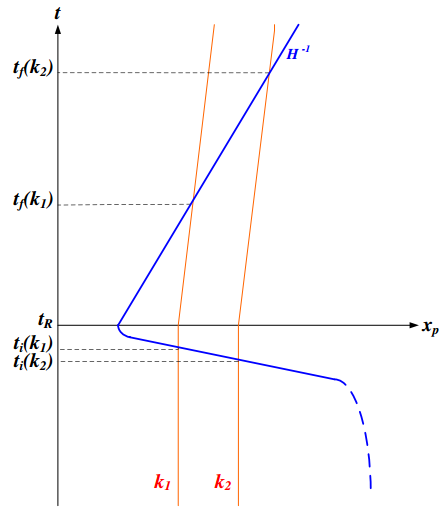Figure 4: Space-time sketch of string gas cosmology. The vertical axis is time, the horizontal one represents physical distance. The early phase $$t < t_R$$$is the stringy Hagedorn phase, during the later phase $$t > t_R$$$ the universe evolves as in Standard Big Bang cosmology. The blue solid curve is the Hubble radius. In the limit that the Hagedorn phase is static, the Hubble radius is actually infinite. During the post Hagedorn phase the Hubble radius increases linearly. The two red curves which are vertical in the Hagedorn phase represent the wavelengths of two fluctuation modes. They exit the Hubble radius at times $$t_i(k)$$$and re-enter at late cosmological times $$t_f(k)$$$.
In Figure 5 we show, for comparison, the corresponding space-time sketch for inflationary cosmology. The axes are as in the previous figure. The time $$t_R$$$denotes the end of the period of inflation. In the case of inflationary cosmology, it is the accelerated expansion of space which leads to the horizon becoming much larger than the Hubble radius, and which leads to the decrease of the Hubble radius in comoving coordinates.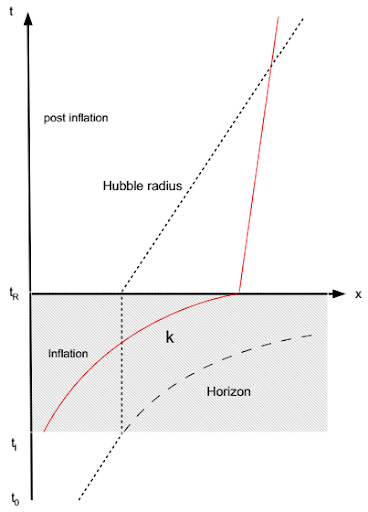Figure 5: Space-time sketch of inflationary cosmology. The vertical axis is time, the horizontal axis represents physical distance. The shaded region ending at time $$t_R$$$ represents the inflationary phase. The dashed line gives the horizon, the dotted line (which overlaps the dashed line in the phase before inflation begins) is the Hubble radius. The red solid curve labelled $$k$$$gives the wavelength of a fluctuation mode. Cosmological Gravitational Waves from String Gas Cosmology In the case of inflationary cosmology, it is postulated that fluctuations of both matter and gravity waves begin as quantum vacuum perturbations on microscopic scales during the period of inflation. When the wavelength of the fluctuations becomes larger than the Hubble radius, the oscillations freeze out, the amplitude of the fluctuations increases and non-linearities lead to a classicalization of the inhomogeneities (Martineau, Kiefer et al.) (see an overview of the theory of cosmological fluctuations). In the limit of perfect exponential expansion of space, the time translation symmetry of the inflationary phase leads to the scale-invariance of the spectrum of perturbations. In the context of matter obeying the standard energy conditions and space-time being described by Einstein's theory of General Relativity, it follows that the spectrum of gravitational waves must have a slight red tilt. The mechanism which leads to the generation of matter fluctuations and gravitational waves in string gas cosmology is completely different. First, during the Hagedorn phase, the state of matter is a dense thermal gas and not a matter vacuum state as in the case of inflation. Hence, in string gas cosmology the fluctuations are thermal in origin. Second, matter is given by a gas of closed strings and not by a gas of point particles. Hence it is string thermodynamics and not point particle thermodynamics which determines the spectrum of the fluctuations which are generated. The spectrum of cosmological fluctuations and gravitational waves predicted by string gas cosmology was worked out about a decade ago in the specific example of a toroidal background (although the general features of the analysis should survive in a more general background). In the case of thermal fluctuations, the cosmological fluctuations with a wavelength $$R$$$ are determined by the specific heat capacity which for a gas of closed strings scales as$\Large \frac{R^2}{ 1 - \frac{T(R)}{T_H}}.$ Here, $$T(R)$$$is the temperature of the string gas when the Hubble radius drops below the scale $$R$$$. Both scalings represent specific stringy prediction for cosmology. The factor $$R^2$$$represents a holographic scaling which can be physically understood by the fact that closed strings in three dimensions look like point particles in one dimension less. It is this holographic scaling which leads to the roughly scale invariance of the spectrum of cosmological fluctuations. The temperature $$T(R)$$$ towards the end of the Hagedorn phase is to a zeroth approximation constant, as Figure 2 shows. However, since $$T(R)$$$decreases slightly as $$R$$$ decreases, the spectrum of cosmological perturbations obtains a slight red tilt, as in the case of inflationary cosmology. The overall amplitude of the spectrum is given by the ratio of the Planck length to the string length, and is thus also a prediction from string theory. As shown here, with the value of the string length preferred on particle physics grounds in the case of heterotic string theory (see e.g. the standard string theory textbook of the 1980s), the amplitude matches the observed one remarkably well.

The spectrum of gravitational waves is given by the anisotropic pressure perturbations in the string gas during the Hagedorn phase. These are also roughly scale invariant. However, since the overall pressure (and hence also the anisotropic pressure fluctuations) are increasing towards the end of the Hagedorn phase, a blue tilt of the spectrum of gravitational waves is induced, in contrast to the case of inflationary cosmology where the decrease in the Hubble rate towards the end of inflation leads inevitably to a slight red tilt $$n_t > 0$$$in the spectrum. The String Gas Cosmology model has two free parameters, the ratio between the Planck length and the string length, and the value of the temperature in the Hagedorn phase. It predicts the four standard observables, namely the amplitudes of the cosmological fluctuations and gravitational waves and the tilts of their spectra $$n_s$$$ and $$n_t$$$. Hence, there are two consistency relations which can be used to test (i.e. rule out) the model. One of them is that a red tilt of the cosmological fluctuations leads to a blue tilt of the gravitational wave spectrum:$n_T \, \simeq \, - (n_s - 1) \, .$ Measurements of the CMB temperature spectrum indicate a value of $$n_s \simeq 0.96$$$ which hence leads to a small predicted blue tilt of the gravitational wave spectrum with $$n_t \simeq 0.04$$\$, which is larger than the limit of sensitivity which future experiments will have. Since the BICEP data gives a hint of a blue spectrum, the String Gas Cosmology consistency relation is slightly preferred over the consistency relation obtained in simple inflationary models.

Conclusions

The discovery of gravitational waves via B-mode polarization of the CMB is a major discovery which is opening a new window to connect fundamental physics with cosmological observations. However, in no way has the discovery confirmed inflationary cosmology, nor has it proven the quantum origin of gravitational waves. The String Gas Cosmology model of early universe cosmology, a model of structure formation alternative to inflation based on fundamental principles of string theory, predicted a spectrum of such gravitational waves. Moreover, it predicted a slight blue tilt of this spectrum, a prediction which at this moment in time seems preferred over the red tilt predicted by inflation. If these data are confirmed by future experiments, cosmology will have verified a quite non-trivial prediction which was first made in the context of string theory.

Acknowledgments

I am grateful to my collaborators Ali Nayeri, Subodh Patil and Cumrun Vafa. In particular, I wish to thank Cumrun for suggestions on the article, and Lubos Motl for the invitation to contribute it.

Written by Robert Brandenberger#### snail feedback (15) :

Dear Robert, thanks for this very interesting essay! I find this whole picture highly intriguing but I still admit to be uncertain whether it may work even at a basic level. I know you're busy but if you had some time to answer a few questions...

1) Does SGC really depend on the T-duality for the thermal circle, or - if this T-duality for the Euclideanized timelike circle were found not to work or work "differently", could SGC be still revived?

2) Should we really interpret the T-duality literally, e.g. by saying that our cold CMB in the Universe around us is equivalent to some super hot trans-Planckian temperature? I remember seeing some problems with the T-duality

3) Does this T-duality really hold non-perturbatively?

4) Don't you overstate the difference between the SGC thermal state and the empty inflating space? The empty inflating spacetime - nearly de Sitter space - is also thermal, isn't it? It has a huge de Sitter entropy and generic states in this ensemble look like the empty de Sitter. Do you see some problems with this picture?

5) Hasn't SGC producing the preferred 3+1 dimensions been kind of diluted or invalidated by the duality revolution in the mid 1990s that placed branes of all dimensions on the same level as fundamental strings? At least naively, the dynamics of various wrapped branes could prefer other spacetime dimensions, couldn't it? What do you think about the result of the string-gas / brane-gas cosmology literature when the dust settled?

6) I've already asked and you told me that they will measure the spectral index for the tensors with the accuracy 0.01. It is a huge precision. Is that really right? Doesn't cosmic variance imply the minimum error in n_T to be of order 0.07 or something like that, as suggested by

http://cosmocoffee.info/viewtopic.php?t=2302
http://arxiv.org/abs/astro-ph/0305411

(if I read the paper correctly and substitute the right numbers etc.)? One question is what are the ambitions of a particular experiment to measure something. But another question where theorists shouldn't rely on experimenters is the fundamental unavoidable error margin caused by cosmic variance. So what is the best precision for "r" and "n_s" and "n_T" that we will be able to measure because of cosmic variance, assuming that n=0.96 or so and r=0.15-0.20 or so? I think that this is a purely theoretical question and the answer for the error margin must be nonzero (positive).Nice application of ficelle. One point for strings!!!!

But is McGill not in qubec.
Where is the French version.
Won't the language police get you for this!!!!

Don't worry I won't tell. :):):):):):)Dear Lubos,

Thanks for your questions. I will attempt very brief replies to them:

1) The T-duality we are using does not involve the Euclidean time circle. It involves only spatial sections. I am told that Calabi-Yau manifolds generally share this T-duality. For example, a quintic threefold has a Z_5 symmetric point which is analogous to the Z_2 T-duality point of the circle.

2) No, this is not the correct interpretation. It would be if we were considering a duality involving Euclidean time.

3) Yes, T-dualities in string theory are exact discrete gauge symmetries and are hence valid beyond perturbative string theory.

4) This question touches a sensitive nerve of mine, but I will be brief: dS has entanglement entropy, not local entropy: when inflation ends, the dS entropy is much less than the entropy after reheating. The entropy which we see now in the universe is produced via nonlinear processes operating during reheating. In SGC, the Hagedorn phase entropy gives the entropy in the SBB phase. Thus, at least in my mind the SGC and inflationary pictures are very different early universe scenarios.

5) Branes can and have been included in string gas cosmology (see e.g. S. Alexander et al, hep-th/0005212). The key point is that higher dimensional branes have an easier time finding each other and hence annihilate first. The critical space-time dimension for p-branes is 2p + 2 = d_c, and hence the branes do not provide any obstruction to 4 space-time dimensions expanding.

6) The information I have is from Matt Dobbs, a colleague of mine at McGill who is a PI on a number of CMB polarization experiments and who is involved in the planning for future experiments. For the value of the tensor to scalar ratio r = 0.2 which BICEP sees, the inflationary consistency relation yields n_t = - 0.04 compared to the tilt of n_t = + 0.04 which the String Gas Cosmology consistency relation yields (using the value n_s = 0.96). Thus, future experiments will be able to differentiate between the predictions of String Gas Cosmology and of standard inflationary models. As Yi Wang and Wei Xue have pointed out in an interesting paper (arXiv:1403.5817), the measurement of non-Gaussianities can be used to differentiate other proposals to get a blue tilted gravitational wave spectrum from that produced by SGC.

7) Although it is nice that the scale-invariance of the spectrum of fluctuations in inflationary cosmology can be seen from the time-translation invariance of physical quantities such as energy density and Hubble radius in the de Sitter phase, inflation is not the only way to obtain a scale-invariant spectrum. In SGC it is the
constancy of the temperature in the quasi-static Hagedorn phase combined with the holographic scaling of the specific heat capacity which combine to yield the scale-invariance of the spectrum. In inflation, fluctuations are of quantum vacuum origin, in SGC they are classical thermodynamic. Thus, it is really very different physics which is at work.

Best wishes, Robert

Thank you for your answer, Robert! The dependence on thermal T-duality would be bad because the Hagedorn temperature isn't even the T-selfdual circumference in bosonic string theory, a factor of 2 is there (probably the most serious error in Joe Polchinski's book that I have found was that he said that they agreed...). In type II, T-duality maps IIA to IIB and vice versa, a different part of the configuration space, so there is no self-duality at all.Thank you for this interesting summary of the string gas cosmology.

A question by an experimentalist::

You say: "However, in no way has the discovery confirmed inflationary cosmology,
nor has it proven the quantum origin of gravitational waves. "

Are the strings postulated in the theory classical strings, not quantized?Dear Prof. Robert Brandenberger, thank you very much by this fascinating post. I maybe have mistaken how SGC can explain the "flatness problem", a well-known success of Inflation. Is there a simple way to explain that? In addition, is it also possible to derive from the SGC scenario why the cosmological constant is so tiny? Thanks beforehand!Inflation does do better at explaining the flatness of the Universe. I grant this. Concerning the cosmological constant problem, SGC offers no explanation for its value. However, in the case of inflationary cosmology the basic mechanism of obtaining exponential expansion of space is not robust against our ignorance of what solves the "old" cosmological constant problem. So here, SGC has an advantage.I should have been a bit more precise and said "quantum vacuum origin". I agree with what you write."in order than [sic] a causal generation mechanism is possible . . ."

I haven't read to the end of this particular post yet but I did read a couple of Robert Brandenberger's earlies papers on the subject back when this story first broke (ht Lubs Motl). Anyway, as an amateur physics buff who does not speak the language of mathematics (in other words a simpleton) I would like to share my impression of string gas cosmologly: it strikes me as intuitively simpler and more plausible than the inflationary theories with their "slow rolls," etc.. In other words, this is an excellent heuristic for rejecting Brandenberger's hypothesis out of hand! (ha ha)Prof. Robert Brandenberger: Thanks for the article on SGC. I did not understand your answer about flatness problem." Inflation does do better at explaining the flatness of the Universe". Do you mean that SGC does explain flatness but the explanation is much more involved or that there is a problem in SGC about explaining flatness? Also what about the monopole problem?Could one say that in these models, the cosmological constant that drives the expansion of space (decompactification of the now large dimension) is mimicked by the subsequently released energy needed (and storred in the winding strings) to keep the expanding dimensions small first?In SGC it is the release of energy stored in winding strings which starts off the expansion of our three spatial dimensions. The cosmological constant does not play a role. We have not addressed the problem of the cosmological constant (why it is not much larger than observed and why it seems to be appearing right at the present time) in our work.In order to address the flatness problem we need to add further inputs. Concerning the monopole problem: given our current understanding of particle physics emerging from string theory we are not starting at very high energy with a simply connected group, and hence monopoles are not inevitable.